Home Practice
For learners and parents For teachers and schools
Textbooks
Full catalogue
Pricing SupportLog in

We think you are located in United States. Is this correct?

# 5.7 Critical angles and total internal reflection

## 5.7 Critical angles and total internal reflection (ESBN9)

### Total internal reflection (ESBNB)

You may have noticed when experimenting with ray boxes and glass blocks in the previous section that sometimes, when you changed the angle of incidence of the light, it was not refracted out into the air, but was reflected back through the block. When the entire incident light ray travelling through an optically denser medium is reflected back at the boundary between that medium and another of lower optical density, instead of passing through and being refracted, this is called total internal reflection.

As we increase the angle of incidence, we reach a point where the angle of refraction is $$\text{90}$$$$\text{°}$$ and the refracted ray travels along the boundary of the two media. This angle of incidence is called the critical angle.

Critical angle

The critical angle is the angle of incidence where the angle of refraction is $$\text{90}$$$$\text{°}$$. The light must travel from an optically more dense medium to an optically less dense medium.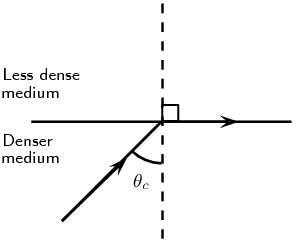Figure 5.15: When the angle of incidence is equal to the critical angle, the angle of refraction is equal to $$\text{90}$$$$\text{°}$$.

If the angle of incidence is bigger than this critical angle, the refracted ray will not emerge from the medium, but will be reflected back into the medium. This is called total internal reflection.

The conditions for total internal reflection are:

1. light is travelling from an optically denser medium (higher refractive index) to an optically less dense medium (lower refractive index).
2. the angle of incidence is greater than the critical angle.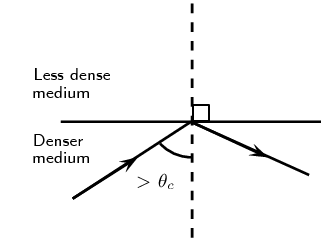Figure 5.16: When the angle of incidence is greater than the critical angle, the light ray is reflected at the boundary of the two media and total internal reflection occurs.

Each pair of media have their own unique critical angle. For example, the critical angle for light moving from glass to air is $$\text{42}$$$$\text{°}$$, and that of water to air is $$\text{48,8}$$$$\text{°}$$.

temp text

A recommended experiment for informal assessment is also included. This covers determining the critical angle for light travelling through a rectangular glass block. Learners will need a rectangular glass block, a $$\text{360}$$$$\text{°}$$ protractor, pencil, paper, ruler and a ray box. Learners should all get similar results at the end of the experiment.

#### Calculating the critical angle

Instead of always having to measure the critical angles of different materials, it is possible to calculate the critical angle at the surface between two media using Snell's Law. To recap, Snell's Law states: $n_1 \sin \theta_1 = n_2 \sin \theta_2$ where $$n_1$$ is the refractive index of material $$\text{1}$$, $$n_2$$ is the refractive index of material $$\text{2}$$, $$\theta_1$$ is the angle of incidence and $$\theta_2$$ is the angle of refraction. For total internal reflection we know that the angle of incidence is the critical angle. So, $\theta_1 = \theta_c.$

However, we also know that the angle of refraction at the critical angle is $$\text{90}$$$$\text{°}$$. So we have: $\theta_2=90^{\circ}.$

We can then write Snell's Law as: $n_1 \sin{\theta_c} = n_2 \sin{90^{\circ}}$

Solving for $$\theta_c$$ gives: \begin{align*} n_1 \sin \theta_c &= n_2 \sin \text{90}\text{°} \\ \sin \theta_c &= \frac{n_2}{n_1}(1) \end{align*} $\boxed{\therefore \theta_c = \sin^{-1}\left(\frac{n_2}{n_1}\right)}$

Remember that for total internal reflection the incident ray is always in the denser medium!

## Worked example 5: Critical angle 1

Given that the refractive indices of air and water are $$\text{1,00}$$ and $$\text{1,33}$$ respectively, find the critical angle.

### Determine how to approach the problem

We can use Snell's law to determine the critical angle since we know that when the angle of incidence equals the critical angle, the angle of refraction is $$\text{90}$$$$\text{°}$$.

### Solve the problem

\begin{align*} n_1 \sin{\theta_c} &= n_2 \sin{90^{\circ}}\\ \theta_c &=\sin^{-1}\left(\frac{n_2}{n_1}\right) \\ &=\sin^{-1}\left(\frac{1}{\text{1,33}}\right) \\ &= \text{48,8}\text{°} \end{align*}

The critical angle for light travelling from water to air is $$\text{48,8}$$$$\text{°}$$.

## Worked example 6: Critical angle 2

Complete the following ray diagrams to show the path of light in each situation.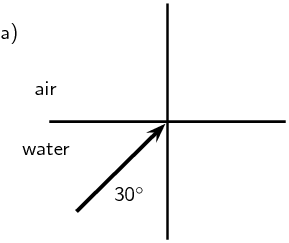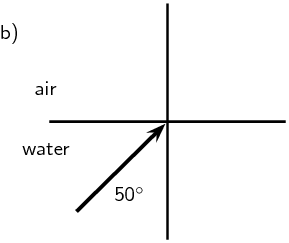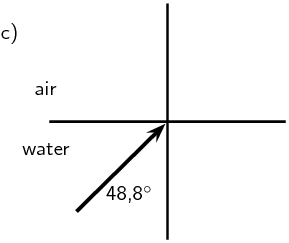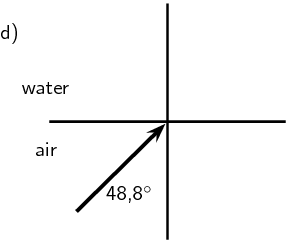### Identify what is given and what is asked

The critical angle for water is $$\text{48,8}$$$$\text{°}$$

We are asked to complete the diagrams.

For incident angles smaller than $$\text{48,8}$$$$\text{°}$$ refraction will occur.

For incident angles greater than $$\text{48,8}$$$$\text{°}$$ total internal reflection will occur.

For incident angles equal to $$\text{48,8}$$$$\text{°}$$ refraction will occur at $$\text{90}$$$$\text{°}$$.

The light must travel from a medium with a higher refractive index (higher optical density) to a medium with lower refractive index (lower optical density).

### Complete the diagrams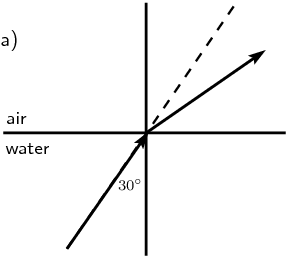Refraction occurs (ray is bent away from the normal).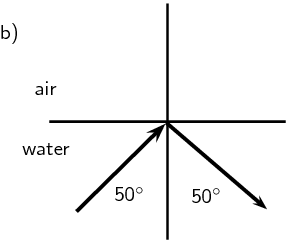Total internal reflection occurs.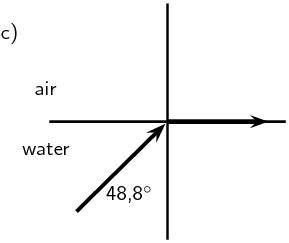$$\theta_c = \text{48,8}\text{°}.$$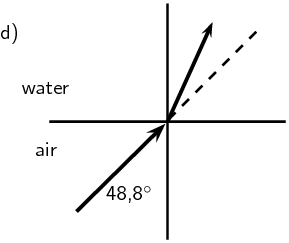Refraction towards the normal (air is less dense than water).

temp text

#### Fibre optics

Total internal reflection is a very useful natural phenomenon since it can be used to confine light. One of the most common applications of total internal reflection is in fibre optics. An optical fibre is a thin, transparent fibre, usually made of glass or plastic, for transmitting light. Optical fibres are usually thinner than a human hair! The construction of a single optical fibre is shown in Figure 5.17.

The basic functional structure of an optical fibre consists of an outer protective cladding and an inner core through which light pulses travel. The overall diameter of the fibre is about $$\text{125}$$ $$\text{μm}$$ ($$\text{125} \times \text{10}^{-\text{6}}$$ $$\text{m}$$) and that of the core is just about $$\text{50}$$ $$\text{μm}$$ ($$\text{50} \times \text{10}^{-\text{6}}$$ $$\text{m}$$). The difference in refractive index of the cladding and the core allows total internal reflection to occur in the same way as happens at an air-water surface. If light is incident on a cable end with an angle of incidence greater than the critical angle then the light will remain trapped inside the glass strand. In this way, light travels very quickly down the length of the cable.

##### Fibre Optics in Telecommunications

Optical fibres are most common in telecommunications, because information can be transported over long distances, with minimal loss of data. This gives optical fibres an advantage over conventional cables.

Signals are transmitted from one end of the fibre to another in the form of laser pulses. A single strand of fibre optic cable is capable of handling over $$\text{3 000}$$ transmissions at the same time which is a huge improvement over the conventional co-axial cables. Multiple signal transmission is achieved by sending individual light pulses at slightly different angles. For example if one of the pulses makes a $$\text{72,23}$$$$\text{°}$$ angle of incidence then a separate pulse can be sent at an angle of $$\text{72,26}$$$$\text{°}$$! The transmitted signal is received almost instantaneously at the other end of the cable since the information coded onto the laser travels at the speed of light! During transmission over long distances repeater stations are used to amplify the signal which has weakened by the time it reaches the station. The amplified signals are then relayed towards their destination and may encounter several other repeater stations on the way.

##### Fibre optics in medicine

Optic fibres are used in medicine in endoscopes.

Endoscopy means to look inside and refers to looking inside the human body for diagnosing medical conditions.

The main part of an endoscope is the optical fibre. Light is shone down the optical fibre and a medical doctor can use the endoscope to look inside the body of a patient. Endoscopes can be used to examine the inside of a patient's stomach, by inserting the endoscope down the patient's throat.

Endoscopes also allow minimally invasive surgery. This means that a person can be diagnosed and treated through a small incision (cut). This has advantages over open surgery because endoscopy is quicker and cheaper and the patient recovers more quickly. The alternative is open surgery which is expensive, requires more time and is more traumatic for the patient.

## Total internal reflection and fibre optics

Textbook Exercise 5.5

Describe total internal reflection by using a diagram and referring to the conditions that must be satisfied for total internal reflection to occur.

If the angle of incidence is bigger than the critical angle, the refracted ray will not emerge from the medium, but will be reflected back into the medium. This is called total internal reflection.

The critical angle occurs when the angle of incidence where the angle of refraction is $$\text{90}$$$$\text{°}$$. The light must travel from an optically more dense medium to an optically less dense medium.

The conditions for total internal reflection are the the light is travelling from an optically denser medium (higher refractive index) to an optically less dense medium (lower refractive index) and that the angle of incidence is greater than the critical angle.

Representing this on a diagram gives: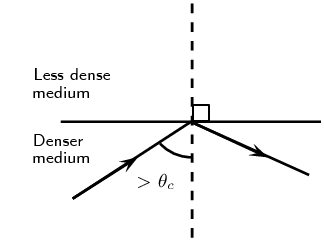Define what is meant by the critical angle when referring to total internal reflection. Include a ray diagram to explain the concept.

The critical angle occurs when the angle of incidence where the angle of refraction is $$\text{90}$$$$\text{°}$$. The light must travel from an optically more dense medium to an optically less dense medium.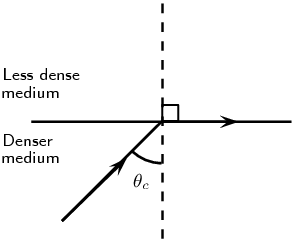Will light travelling from diamond to silicon ever undergo total internal reflection?

Diamond (index of refraction is about 3) is less optically dense than silicon (index of refraction is about 4) and so total internal reflection cannot occur.

Will light travelling from sapphire to diamond undergo total internal reflection?

Sapphire (index of refraction is $$\text{1,77}$$) is less optically dense than diamond (index of refraction is about 3) and so total internal reflection cannot occur.

What is the critical angle for light travelling from air to acetone?

The critical angle is:

\begin{align*} n_1 \sin{\theta_c} &= n_2 \sin{90^{\circ}}\\ \theta_c &=\sin^{-1}\left(\frac{n_2}{n_1}\right) \\ &=\sin^{-1}\left(\frac{1}{\text{1,36}}\right) \\ &= \text{47,33}\text{°} \end{align*}

Light travelling from diamond to water strikes the interface with an angle of incidence of $$\text{86}$$$$\text{°}$$ as shown in the picture. Calculate the critical angle to determine whether the light be totally internally reflected and so be trapped within the diamond.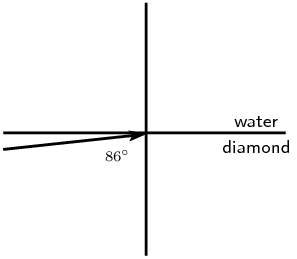The critical angle is:

\begin{align*} n_1 \sin{\theta_c} &= n_2 \sin{90^{\circ}}\\ \theta_c &=\sin^{-1}\left(\frac{n_2}{n_1}\right) \\ &=\sin^{-1}\left(\frac{\text{1,33}}{\text{2,419}}\right) \\ &= \text{33,35}\text{°} \end{align*}

The angle of incidence is greater than the critical angle and so the light will be trapped within the diamond.

Which of the following interfaces will have the largest critical angle?

1. a glass to water interface
2. a diamond to water interface
3. a diamond to glass interface
a glass to water interface

If a fibre optic strand is made from glass, determine the critical angle of the light ray so that the ray stays within the fibre optic strand.

The critical angle is:

\begin{align*} n_1 \sin{\theta_c} &= n_2 \sin{90^{\circ}}\\ \theta_c &=\sin^{-1}\left(\frac{n_2}{n_1}\right) \\ &=\sin^{-1}\left(\frac{\text{1}}{\text{1,5}}\right) \\ &= \text{41,8}\text{°} \end{align*}

A glass slab is inserted in a tank of water. If the refractive index of water is $$\text{1,33}$$ and that of glass is $$\text{1,5}$$, find the critical angle.

The critical angle is:

\begin{align*} n_1 \sin{\theta_c} &= n_2 \sin{90^{\circ}}\\ \theta_c &=\sin^{-1}\left(\frac{n_2}{n_1}\right) \\ &=\sin^{-1}\left(\frac{\text{1,33}}{\text{1,5}}\right) \\ &= \text{62,46}\text{°} \end{align*}

Note that the light must be travelling from the glass into the water for total internal reflection to occur.

A diamond ring is placed in a container full of glycerin. If the critical angle is found to be $$\text{37,4}$$$$\text{°}$$ and the refractive index of glycerin is given to be $$\text{1,47}$$, find the refractive index of diamond.

The refractive index is:

\begin{align*} n_1 \sin{\theta_c} &= n_2 \sin{90^{\circ}}\\ n_{1} &= \frac{n_1}{\sin{\theta_c}} \\ &= \frac{\text{1,47}}{ \sin \text{37,4}\text{°}} \\ &= \text{2,42}\text{°} \end{align*}

An optical fibre is made up of a core of refractive index $$\text{1,9}$$, while the refractive index of the cladding is $$\text{1,5}$$. Calculate the maximum angle which a light pulse can make with the wall of the core. NOTE: The question does not ask for the angle of incidence but for the angle made by the ray with the wall of the core, which will be equal to $$\text{90}$$$$\text{°}$$ − angle of incidence.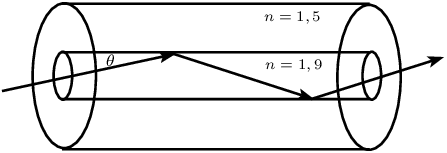The critical angle is:

\begin{align*} n_1 \sin{\theta_c} &= n_2 \sin{90^{\circ}}\\ \theta_c &=\sin^{-1}\left(\frac{n_2}{n_1}\right) \\ &=\sin^{-1}\left(\frac{\text{1,5}}{\text{1,9}}\right) \\ &= \text{52,14}\text{°} \end{align*}

The maximum angle is: $$\text{90}\text{°} - \text{52,14}\text{°} = \text{37,86}\text{°}$$